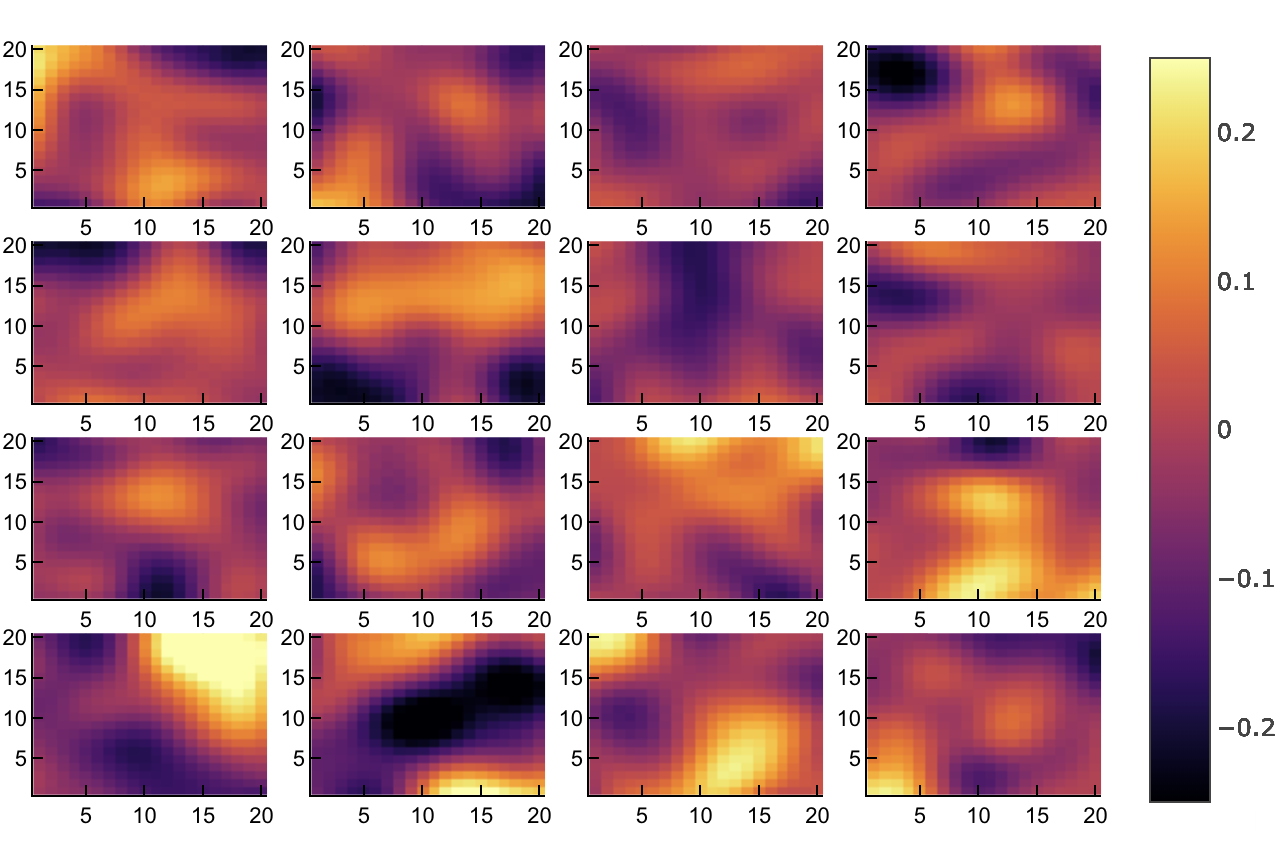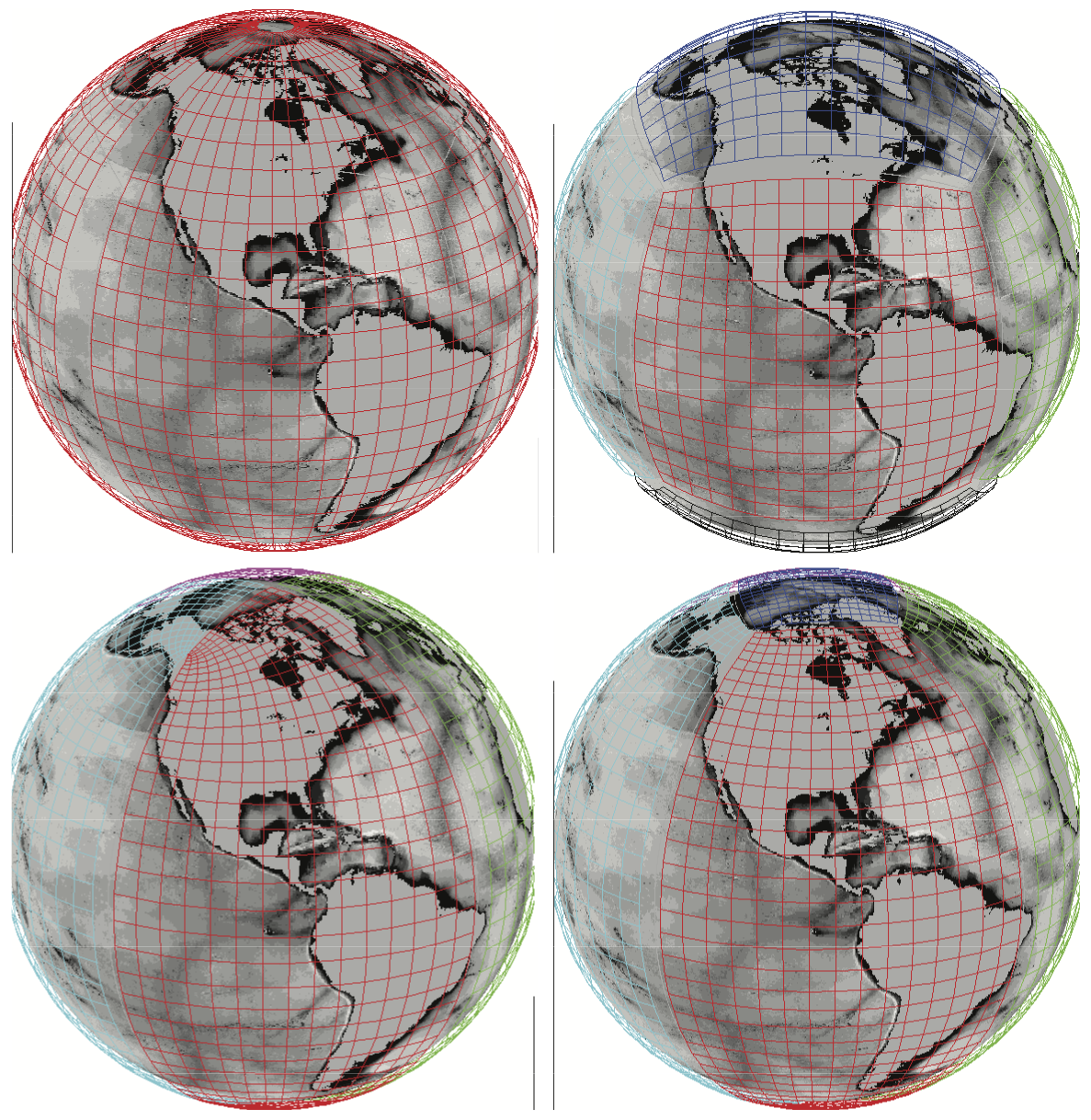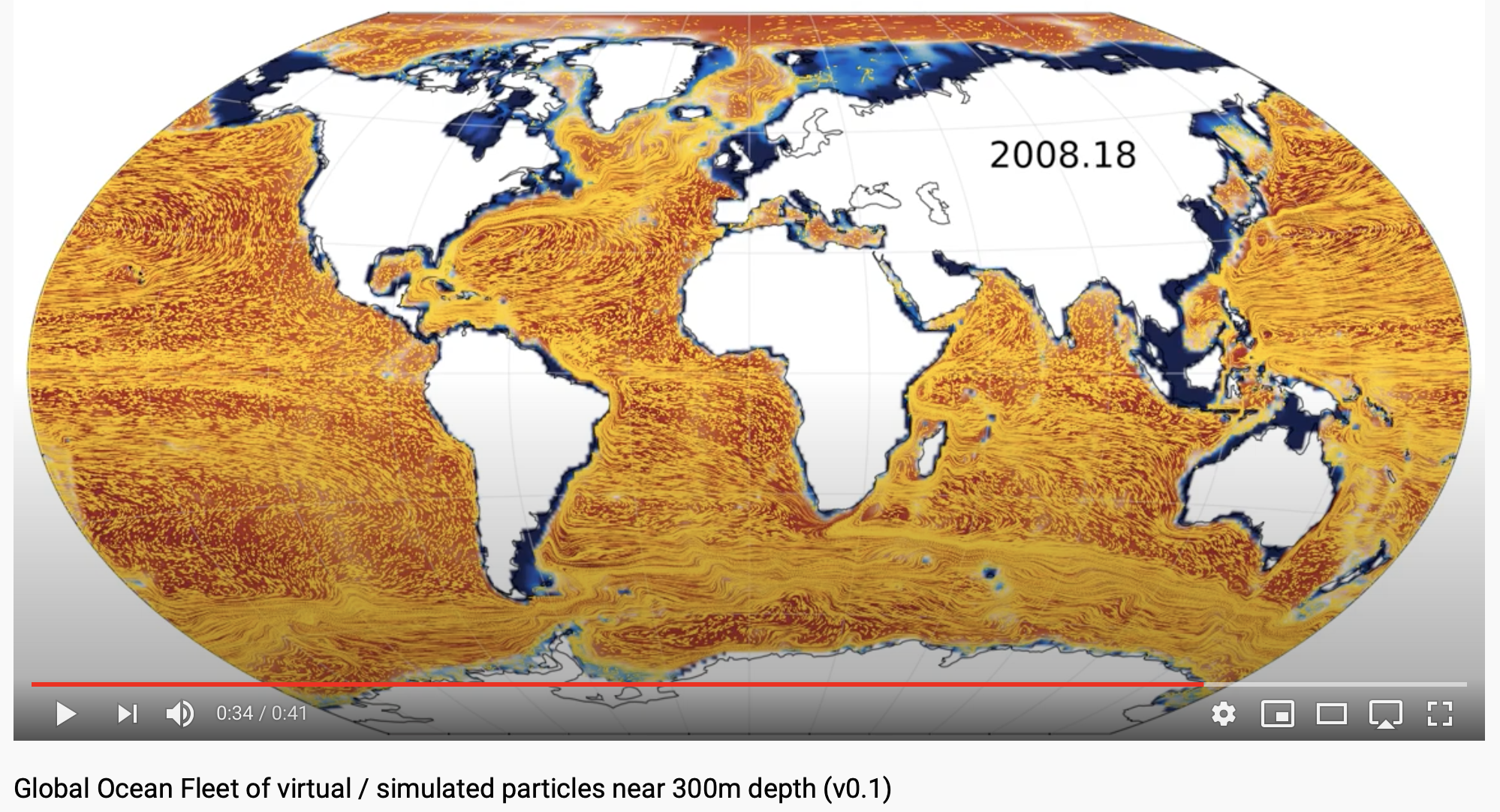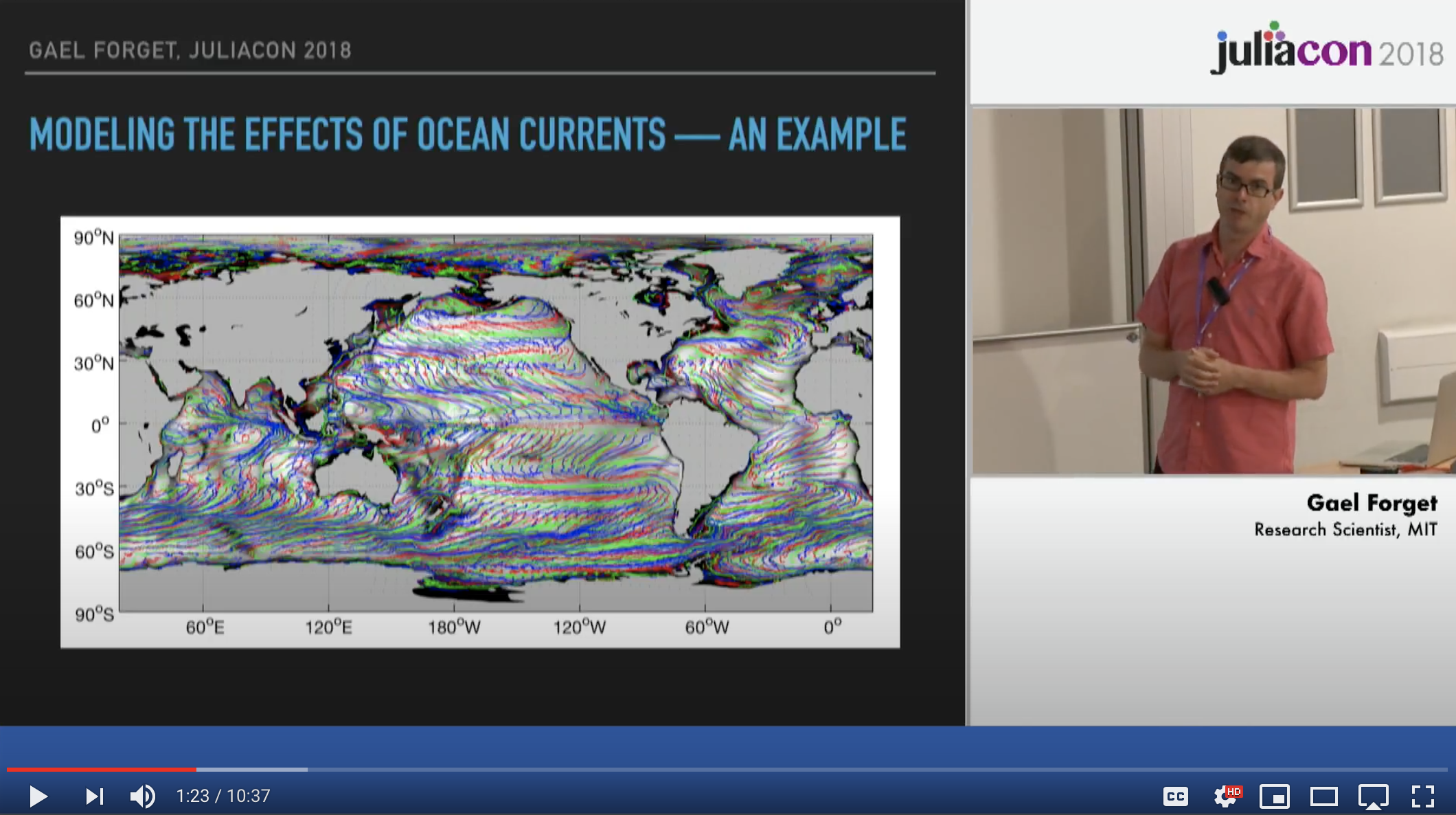0

0

0

4

# MeshArrays.jl

`MeshArrays.jl` defines the `MeshArray` type that can contain, and distribute, collections of inter-connected arrays as generally done in climate models. This provides a simple yet efficient and general way to e.g. analyze climate system simulations.

``````struct gcmarray{T, N} <: AbstractMeshArray{T, N}
grid::gcmgrid
meta::varmeta
f::Array{Array{T,2},N}
fSize::Array{NTuple{2, Int}}
fIndex::Array{Int,1}
version::String
end
``````

### Installation

``````using Pkg
Pkg.test("MeshArrays")
``````

### Use Examples

The example below (1) generates a grid decomposition, (2) seeds random noise everywhere, (3) smoothes out the noise, and (4) plots the (`outer`) array of subdomain (`inner`) arrays. The diffusion-based smoother illustrates how `MeshArrays.jl` computes partial derivatives over the whole domain by transfering data between neighboring subdomains.

``````using MeshArrays; p=dirname(pathof(MeshArrays))
γ,Γ=GridOfOnes("PeriodicDomain",16,20)

include(joinpath(p,"../examples/Demos.jl"))
(xi,xo,_,_)=demo2(Γ);
show(xo)

using Plots; plotlyjs()
include(joinpath(p,"../examples/Plots.jl"))
heatmap(xo,clims=(-0.25,0.25))
``````Above, we used 16 subdomains, with 40x40 grid points each, covering a standard doubly periodic domain. However, `MeshArrays.jl` also readily supports elaborate grids commonly used in climate models, such as the ones shown below.### Jupyter Notebooks

The Global Ocean Notebooks illustrate:

• Using `MeshArrays.jl` to accurately compute planetary transports on a ocean model C-grid.
• Using `MeshArrays.jl` with IndividualDisplacements.jl to efficiently compute trajectories of ocean plastic, plankton, etc over the C-grid configurations supported by `MeshArrays.jl`.
• Support for CF-compliant Netcdf input / output of `MeshArray`s, with interpolation or domain decomposition, for `C-grid` variables as provided via NCTiles.jl.
• Support for MITgcm use cases and specificities is provided via MITgcmTools.jl.(Jupyter notebook docs)

### JuliaCon 2018 Videowhere `MeshArrays.jl` was first introduced as as `gcmfaces.jl`. The presentation corresponds to GlobalOceanNotebooks `/DataStructures/01_MeshArrays.ipynb`

06/17/2020

11 months ago

155 commits